#### Diffraction Limited Mode

In diffraction limited mode with adaptive optics the PSF is approximately composed of two functions:
1. The core: This is an airy function of the telescope

 IAiry(r)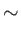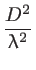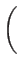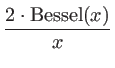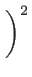(6)

 D : diameter of the telescope mirror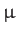: observing wavelength Bessel : the the first kind Bessel function of the order 1.
2. The halo: It is given by a Moffat function

 IMoffat(r) = I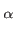,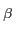(r) =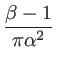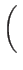1 +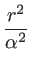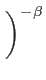(7)

 I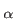,(r) : Intensity at the distance r with parameters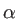and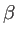: Parameter is used to fix the FWHM for a given: Parameter to fix the amount of light in the lobes r : Distance to the center (r =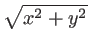) FWHM : 2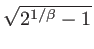An example of such combined PSF is shown in Figure 1.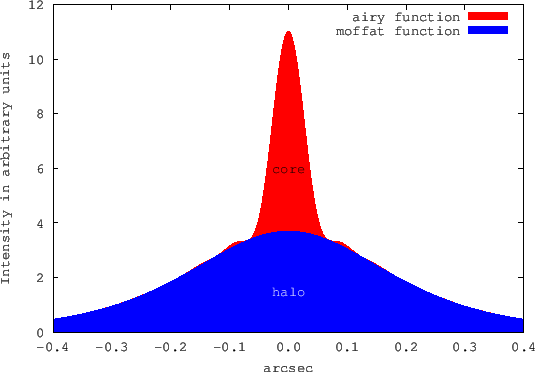For comparing the peak intensity of an ideal diffraction-limited optical system with a real system the Strehl parameter was introduced.

 Strehl =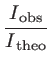(8)

This parameter is the ratio of the observed peak intensity at the detection plane of a telescope or other imaging system from a point source compared to the theoretical maximum peak intensity of a perfect imaging system working at the diffraction limit. For calculating the fraction of the halo and core component to achieve a certain strehl ratio the parameter F0 is introduced in this ETC. It has to fulfill the following equation:

 Strehl ==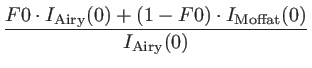(9)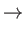F0 =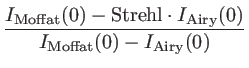(10)

In this mode the SNR is calculated for a disk with a radius of twice the radius of the airy disk.

Andre Germeroth 2016-11-04